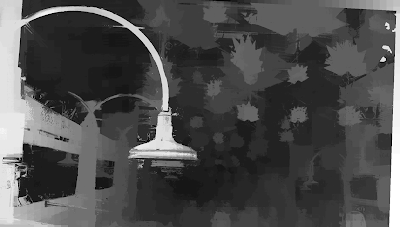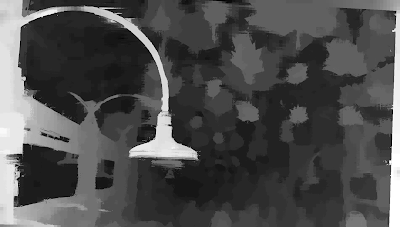## Thursday, September 28, 2017

### Case Study - DMAG5+DMAG9b

We are gonna use John Hooper's "People Waiting" in Saint-John, New Brunswick, to illustrate what DMAG9b can do to a depth map produced by DMAG5.

Sometime, ER9b is a bit too aggressive when rectifying and you end up with quite large camera rotations (resulting in a zooming effect). In those cases, I simply switch to ER9c, like in this case.

Let's get a "basic" depth map using DMAG5.

I used the following parameters in DMAG5:
alpha = 0.9
truncation (color) = 30
epsilon = 255^2*10^-4
disparity tolerance = 0
radius to smooth occlusions = 9
sigma_space = 9
sigma_color = 25.5
downsampling factor = 2

One could play around with the radius and epsilon to perhaps get a better depth map, but it's simpler to just let DMAG9b operate its magic.

I used the following parameters in DMAG9b:
sample_rate_spatial = 32
sample_rate_range = 8
lambda = 0.25
hash_table_size = 100000
nbr of iterations (linear solver) = 25
sigma_gm = 1
nbr of iterations (irls) = 32
gamma proximity (confidence map) = 12
gamma color similarity (confidence map) = 12
sigma (confidence map) = 32

Let's change the spatial sample rate from 32 to 16, leaving everything else as is, and let's rerun DMAG9b.

Let's change the spatial sample rate from 16 to 8, leaving everything else as is, and let's rerun DMAG9b.

The depth map looks pretty good, so we are gonna stop here.

### Case Study - DMAG5b+DMAG9b

The main parameter to tinker with when using DMAG5b is the radius. The lower the radius, the more noise. The larger the radius, the smoother the depth map is gonna be but the more inaccurate at object boundaries.

Let's stick with that depth map (for no particular reason) and post-process it with DMAG9b. The main parameters to tinker with in DMAG9b are the two sample rates (space and range aka color) and lambda (the smoothing multiplier).Depth map obtained by DMAG5b using radius = 16 and post-processed by DMAG9b using sample_rate_spatial = 32, sample_rate_range = 8, and lambda = 0.25.

What happens if you decrease the spatial sample rate from 32 to 16?Depth map obtained by DMAG5b using radius = 16 and post-processed by DMAG9b using sample_rate_spatial = 16, sample_rate_range = 8, and lambda = 0.25.

I think it's a bit better. I tried decreasing the range (color) sample rate from 8 to 4 but there was no improvement.

What happens if you increase lambda from 0.25 to 1.0?Depth map obtained by DMAG5b using radius = 16 and post-processed by DMAG9b using sample_rate_spatial = 16, sample_rate_range = 8, and lambda = 1.0.

Maybe it's a bit better although debatable. I tried to go the other way and decrease lambda but there was no improvement. So, let's call it a day and stop here.

## Wednesday, September 27, 2017

### 3D Image Conversion - Trinity

We are gonna use DMAG4 to semi-automatically convert a 2d image (still from "The Matrix" movie) into 3d via a depth map.

The "edge image" is simply a red trace (1 pixel wide) over the object boundaries. It takes about 5 minutes to do with the "Paths Tool". The "edge image" indicates depth changes and drastically simplifies the task of the dense depth map generator DMAG4. In the sparse depth map, I make use of the "Gradient Tool" when I need to establish depths along a slanted plane, here, along ground and gun. Otherwise, I just use the "Pencil Tool" ... very sparingly. I don't have to use the pencil much because of the presence of the "edge image".

I used the following parameters for DMAG4:
beta = 10
maxiter = 5000
scale_nbr = 1
con_level = 1
con_level2 = 1

Note the beta I am using. It's rather small. This makes DMAG4 kinda like an isotropic diffuser (of depths).

In wigglemaker, I chose "None" for inpainting method. I think it works best. Let me know if you don't think so! If you insist on relatively proper inpainting, it's probably a good idea to reduce the camera offset.

## Wednesday, September 6, 2017

### 3D Image Conversion - Top Gun

In this post, we are gonna look at 2d to 3d image conversion using DMAG4.

As you probably know, the input to DMAG4 is the 2d image, a sparse depth map, and possibly what I call an "edge image". The purpose of the edge image is to separate different objects in the scene. DMAG4 will not propagate depths past an edge in the edge image, which means you don't have to worry about the beta parameter.

I drew the "edge image" in Gimp using the "Paths" tool and stroking the path once done. Use the "Stroke Line" option, turn the anti-aliasing off, and choose 1 pixel for the width when stroking the path. It takes about 5 minutes to get the "edge image". Because I use an "edge image", DMAG4 can be allowed to propagate regardless of color similarity and therefore it is ok to use a small beta (makes the bilateral filter in DMAG4 behave like a regular Gaussian filter). Having an "edge image" makes things much easier when it is time to draw the sparse depth map and give the depth clues to DMAG4.

I took a pretty minimalist approach when drawing the sparse depth map, which means it is very sparse. To draw the sparse depth map in Gimp, I use the "Pencil" tool with an hard edge brush (no anti-aliasing). It takes another 5 minutes to draw the sparse depth map. When creating the sparse depth map, the important thing is the relationship between the various depths, not the actual depths. Make sure that when you zoom on the sparse depth map brushed on areas, the edges look jagged, which means there is no anti-aliasing applied, which is what we want.

We now have everything we need to launch DMAG4 and get the dense depth map.

I used the following parameters in DMAG4:
beta = 10
maxiter = 5000
scale_nbr = 1
con_level = 1
con_level2 = 1

Again, because I used an "edge image", I really don't have to worry about depths propagating across objects. This means that I can use a low beta (here, equal to 10). When beta is low, DMAG4 behaves pretty much like a classic Gaussian filter. If beta is large, DMAG4 behaves like a bilateral filter, in other words, it propagates depths only along similar colors, which can be a real problem in some cases. The idea behind using a bilateral filter is that things that are not of the same color probably don't belong to the same object and are probably at different depths. Of course, this is not ideal because you can clearly have different colors within an object. Because of that, if you don't use an "edge image", the sparse depth map may need to be not so sparse at all and a lot of time is wasted drawing the sparse depth map, running DMAG4, and fixing the sparse depth map before going through another iteration. Conclusion: use an "edge image" and a low beta!